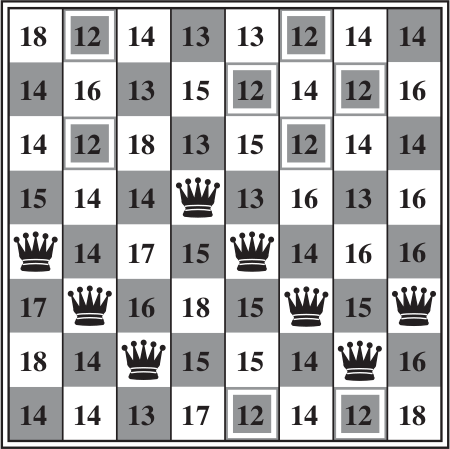# 8queens_tutorial

Manlio Morini edited this page Feb 11, 2019 · 9 revisions

# Eight Queens Puzzle with Genetic AlgorithmsThe eight queens puzzle is the problem of placing eight chess Queens on an 8×8 chessboard so that no two queens threaten each other; thus, a solution requires that no two queens share the same row, column, or diagonal.

NOTE. The following example is derived from Artificial Intelligence: A Modern Approach.

## Individuals

Local search algorithms typically use a complete-state formulation, where each state has 8 queens on the board, one per column. The successors of a state are all possible states generated by moving a single queen to another square in the same column.

Classical GAs represent a state (here called chromosome or individual) with a string of `0`s / `1`s (it requires 24 bits to specify the position of 8 queens each in a column of 8 squares).

Alternatively each individual can represented as a an eight-numbers (in the `[1,8]` interval) vector.

We will see that these two encodings behave differently. In Vita we adopt the second representation (with the difference that, as usual in C/C++, the interval is `[0,7]` instead of `[1,8]`):

```const int NQUEENS(8);

vita::ga_problem prob(NQUEENS, {0, NQUEENS});```

which is a short cut for:

```const int NQUEENS(8);

vita::ga_problem prob;

for (int i(0): i < NQUEENS; ++i)
prob.insert(range(0, 8));```

## Fitness function

The starting point is the heuristic cost function `h`: the number of pairs of queens that are attacking each other, either directly or indirectly.

The global minimum of this function is 0, which occurs only at perfect solutions.Figure shows a state with `h = 17`. The figure also shows the values of all its successors, with the best successors having `h = 12`.

A fitness function should return higher values for better states, so for this task we use `f = -h` (in general minimizing a function `g` is equivalent to maximizing `-g`).## Highlights

##### Clone this wiki locally
You can’t perform that action at this time.